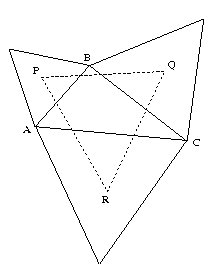#### You may also like### LOGO Challenge 5 - Patch

Using LOGO, can you construct elegant procedures that will draw this family of 'floor coverings'?### LOGO Challenge - Triangles-squares-stars

Can you recreate these designs? What are the basic units? What movement is required between each unit? Some elegant use of procedures will help - variables not essential.### LOGO Challenge - Tilings

Three examples of particular tilings of the plane, namely those where - NOT all corners of the tile are vertices of the tiling. You might like to produce an elegant program to replicate one or all of these.

# Napoleon's Theorem

##### Age 14 to 18 Challenge Level:Triangle $ABC$ has equilateral triangles drawn on its edges. Points $P$, $Q$ and $R$ are the centres of the equilateral triangles. Experimentation with the interactive diagram leads to the conjecture that $PQR$ is an equilateral triangle. There are many ways to prove this result. Here we have chosen two methods, one which uses only the cosine rule and one which uses complex numbers to represent vectors, and multiplication by complex numbers to rotate the vectors by 60 degrees.

Another proof using a tessellation of the plane is discussed on the Cut-the-knot website.

First the proof using the Cosine Rule.
The sides of triangle $ABC$ are written as $a, b$ and $c$. Centroids of equilateral triangles are at the intersection of the altitudes so $\angle PAB$ and $\angle RAC$ are both 30 degrees. Hence

$$AP = {2\over 3}.{\sqrt 3 c\over 2}= {c\over \sqrt 3}$$ and $$AR = {2\over 3}.{\sqrt 3 b\over 2}= {b\over \sqrt 3}.$$

It follows that $\angle PAR = (\angle A + 60)$ degrees. By the cosine rule

$$PR^2 = AP^2 + AR^2 - 2AP.AR \cos (\angle A+60) = {1\over 3}(c^2 + b^2 - 2bc \cos (\angle A + 60) \quad (1).$$

Now $\cos (\angle A + 60) = {1\over 2}\cos A - {\sqrt 3\over 2}\sin A$ and, from $\triangle ABC$: $\cos A = {b^2 + c^2 - a^2 \over 2bc}$ and $\sin A = {2{\rm Area}\triangle ABC\over bc}$. Substituting for $\cos (\angle A + 60)$ in (1) and simplifying the expression gives:

$$PR^2 = {1\over 3}\left[{a^2 + b^2 + c^2\over 2} + 2\sqrt 3 {\rm Area}\triangle ABC\right].$$

This formula is completely symmetric in $a, b$ and $c$ and it follows that $RQ^2$ and $QP^2$ have the same value and that $\triangle PQR$ is equilateral.

Next the proof using complex numbers as vectors.
We use $\lambda = e^{\pi i/3}$ so that $\lambda ^2 = \lambda - 1$.

Also multiplying a complex number by $\lambda$ rotates it by 60 degrees.

Referring to the given diagram let $A, B, C$ be represented by the complex numbers $a, b, c$. The third vertex of the equilateral triangle drawn on $AB$ is represented by the complex number $a+ \lambda (b-a)$. Therefore the centre of this triangle P is represented by $p$ where

$$p = {1\over 3}([2 - \lambda ]a +[1 +\lambda ]b).$$ Similarly $$q = {1\over 3}(2 - \lambda ]b +[1 + \lambda ]c),$$

and

$$r = {1\over 3}(2 - \lambda ]c +[1 + \lambda ]a).$$

To show that $PQR$ is equilateral it is sufficient to show that $r - q = \lambda [p - q]$ and this follows using simple algebra and $\lambda ^ 2 = \lambda - 1$.